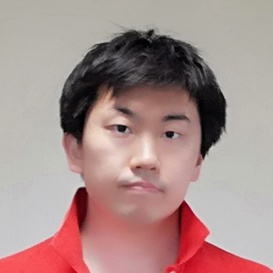atcoder

# # Problem

## # Input

$c_1 c_2 ... c_{10}$
$c_{11} c_{12} ... c_{20}$
...
$c_{91} c_{92} ... c_{100}$.

• $c_i$ - Either x or o.

## # Output

Report if all the o are connected as 4-neighbor (up, down, left or right).
You can flip a x to o.

# # Time complexity

$O(H W)$ for bit exhaustive search.
And $O(H W)$ for DFS for each step in bit exhaustive search.

$O((H W)^2)$

# # Solution

#define MAX_N 10

Int H, W;
vector<bool> C(MAX_N*MAX_N, true);

void input() {
char x;
H = W = 10;
loop(h,0,H) {
loop(w,0,W) {
cin >> x;
if (x == 'x') C[h*W + w] = false;
}
}
}

void dfs(Int x, Int y, Int depth, vector<bool> &c) {
c[y*W+x] = false;
loop(dy,-1,2) {
loop(dx,-1,2) {
if (dy != 0 && dx != 0) continue;
Int x_ = x + dx, y_ = y + dy;
if (x_ < 0 || W <= x_ || y_ < 0 || H <= y_) continue;
if (!c[y_*W + x_]) continue;
dfs(x_, y_, depth+1, c);
}
}
}

bool bit(Int x, Int y, vector<bool> c) {
c[y*W+x] = true;
dfs(x, y, 0, c);
loop(h,0,H) {
loop(w,0,W) {
if (c[h*W+w]) return false;
}
}

return true;
}

void solve() {
loop(h,0,H) {
loop(w,0,W) {
if (bit(w, h, C)) {
cout << "YES" << endl;
return;
}
}
}

cout << "NO" << endl;
}

int main(void) {
input();
solve();
return 0;
}Remote freelancer. A web and mobile application enginner.
Traveling around the world based on East Asia.
I'm looking forward to your job offers from all over the world!

Offer jobs or contact me!